# PyData Global Sprint

## PyTorch-Ignite

High-level library to help with training and evaluating neural networks in PyTorch flexibly and transparently.

.
``````if __name__ == "__main__":
print("Let's have fun helping PyTorch-Ignite open source project !")
``````
.Priyansi @Priyansi A CS Undergrad. Currently working on the docs of PyTorch-Igniteand helping manage the communityJeff Yang@ydcjeff Contributor to PyTorch-Ignite and its related projectsAhmed @KickItLikeShika A CS Undergrad and a Machine Learning Intern at Factmata working on NLPand contributor to PyTorch-Ignite.Victor @vfdev-5 Software Engineer at Quansight working on AI-related open source projects

# Content

2. What are PyTorch and PyTorch-Ignite ?
3. Quick-start PyTorch-Ignite example
4. How you can help ?

Community-driven open source and NumFOCUS Affiliated Project

maintained by volunteers in the PyTorch community:

``````@vfdev-5, @ydcjeff, @KickItLikeShika, @sdesrozis, @alykhantejani, @anmolsjoshi,
@trsvchn, @fco-dv, @Priyansi, @Moh-Yakoub, @gucifer, @Ishan-Kumar2 ...
``````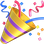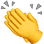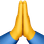With the support of: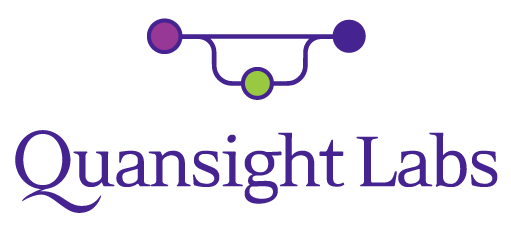# Community Engagement

• Google Summer of Code 2021

• Mentored two great students (Ahmed and Arpan)
• Google Season of Docs 2021

• Working with great tech writer (Priyansi)
• Hacktoberfest 2020 and 2021

• PyData Global Mentored Sprint 2020 and 2021

• Public meetings on Discord, open to everyone

Stay tuned for upcoming events …# PyTorch in a nutshell

 ``````import torch import torch.nn as nn device = "cuda" class MyNN(nn.Module): def __init__(self): super(MyNN, self).__init__() self.flatten = nn.Flatten() self.linear_relu_stack = nn.Sequential( nn.Linear(28*28, 512), nn.ReLU(), nn.Linear(512, 512), nn.ReLU(), nn.Linear(512, 10) ) def forward(self, x): x = self.flatten(x) logits = self.linear_relu_stack(x) return logits model = MyNN().to(device) `````` .tensor manipulations (device: CPUs, GPUs, TPUs)NN components, optimizers, loss functionsDistributed computationsProfilingother cool features …Domain libraries: vision, text, audioRich ecosystem

https://pytorch.org/tutorials/beginner/basics/intro.html

# Quick-start ML with PyTorch

Computer Vision example with Fashion MNIST

Problem: 1 - how to classify images ?

`model(image) -> predicted label`

2 - How measure model performances ?

`predicted labels vs correct labels`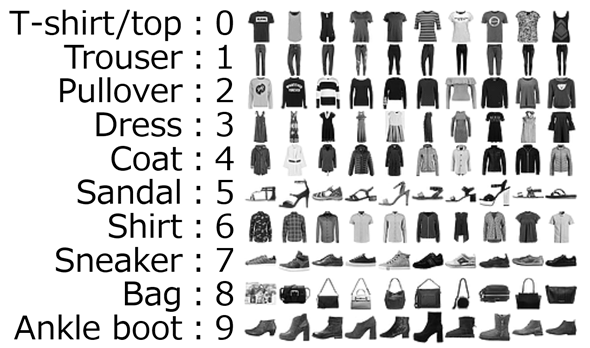# Quick-start ML with PyTorch

• Setup training and testing data
``````from torch.utils.data import DataLoader
from torchvision import datasets
from torchvision.transforms import ToTensor, Lambda, Compose

# Setup training/test data
test_data = datasets.FashionMNIST(root="data", train=False, transform=ToTensor())

batch_size = 64

# Optionally, for debugging:
print("Shape of X [N, C, H, W]: ", X.shape)
print("Shape of y: ", y.shape, y.dtype)
break

# Output:
# Shape of X [N, C, H, W]:  torch.Size([64, 1, 28, 28])
# Shape of y:  torch.Size() torch.int64
``````

# Quick-start ML with PyTorch

• Create a model
``````import torch
from torch import nn

device = "cuda" if torch.cuda.is_available() else "cpu"

class NeuralNetwork(nn.Module):
def __init__(self):
super(NeuralNetwork, self).__init__()
self.flatten = nn.Flatten()
self.linear_relu_stack = nn.Sequential(
nn.Linear(28*28, 512),
nn.ReLU(),
nn.Linear(512, 512),
nn.ReLU(),
nn.Linear(512, 10)
)

def forward(self, x):
x = self.flatten(x)
logits = self.linear_relu_stack(x)
return logits

model = NeuralNetwork().to(device)
``````

# Quick-start ML with PyTorch

• Model training
• Loss function: cross-entropy
• Optimization with Stochastic Gradient Descent
``````loss_fn = nn.CrossEntropyLoss()
optimizer = torch.optim.SGD(model.parameters(), lr=1e-3)

model.train()
X, y = X.to(device), y.to(device)
pred = model(X)
loss = loss_fn(pred, y)

# Backpropagation
loss.backward()
optimizer.step()

# code to compute and print average loss and accuracy

epochs = 5
for t in range(epochs):
print(f"Epoch {t+1}\n-------------------------------")
print("Done!")
``````

## Why using PyTorch without Ignite is suboptimal ?

For NN training and evaluation:

• PyTorch gives only “low”-level building components
• Common bricks to code in any user project:
• metrics
• checkpointing, best model saving, early stopping, …
• logging to experiment tracking systems
• code adaptation for device (e.g. GPU, XLA)
• Pure PyTorch code
``````
model = Net()
optimizer = torch.optim.SGD(model.parameters(), lr=0.01, momentum=0.8)
criterion = torch.nn.NLLLoss()

max_epochs = 10
validate_every = 100
checkpoint_every = 100

model = model.eval()
num_correct = 0
num_examples = 0
input, target = batch
output = model(input)
correct = torch.eq(torch.round(output).type(target.type()), target).view(-1)
num_correct += torch.sum(correct).item()
num_examples += correct.shape
return num_correct / num_examples

def checkpoint(model, optimizer, checkpoint_dir):
# ...

def save_best_model(model, current_accuracy, best_accuracy):
# ...

iteration = 0
best_accuracy = 0.0

for epoch in range(max_epochs):
model = model.train()
input, target = batch
output = model(input)
loss = criterion(output, target)
loss.backward()
optimizer.step()

if iteration % validate_every == 0:
print("After {} iterations, binary accuracy = {:.2f}"
.format(iteration, binary_accuracy))
save_best_model(model, binary_accuracy, best_accuracy)

if iteration % checkpoint_every == 0:
checkpoint(model, optimizer, checkpoint_dir)
iteration += 1

``````

# PyTorch-Ignite: what and why? 🤔

High-level library to help with training and evaluating neural networks in PyTorch flexibly and transparently.

 `````` def train_step(engine, batch): # ... any training logic ... return batch_loss trainer = Engine(train_step) # Compose your pipeline ... trainer.run(train_loader, max_epochs=100) `````` `````` metrics = { "precision": Precision(), "recall": Recall() } evaluator = create_supervised_evaluator( model, metrics=metrics ) `````` ``````@trainer.on(Events.EPOCH_COMPLETED) def run_evaluation(): evaluator.run(test_loader) handler = ModelCheckpoint( '/tmp/models', 'checkpoint' ) trainer.add_event_handler( Events.EPOCH_COMPLETED, handler, {'model': model} ) ``````

# Key concepts in a nutshell

1. Engine and Event System
2. Out-of-the-box metrics to easily evaluate models
3. Built-in handlers to compose training pipeline
4. Distributed Training support

# What makes PyTorch-Ignite unique ?

• Composable and interoperable components
• Simple and understandable code
• Open-source community involvement

# How PyTorch-Ignite makes user’s live easier ?

With PyTorch-Ignite:

• Less code than pure PyTorch while ensuring maximum control and simplicity
• Easily get more refactored and structured code
• Extensible API for metrics, experiment managers, and other components
• Same code for non-distributed and distributed configs

# Quick-start PyTorch-Ignite example 👩‍💻👨‍💻

Let’s train a MNIST classifier with PyTorch-Ignite!

.

https://pytorch-ignite.ai/tutorials/beginner/01-getting-started/

Any questions before we go on ?

# How you can help ?

Participating GitHub repositories:

# Prerequisites

• Basic Python knowledge
• Basic PyTorch knowledge
• Basic Machine Learning knowledge

# Start contributing

## Codebase structure

• ignite - Core library files
• engine - Module containing core classes like Engine, Events, State.
• handlers - Module containing out-of-the-box handlers
• metrics - Module containing out-of-the-box metrics
• contrib - Contrib module with other metrics, handlers classes that may require additional dependencies
• distributed - Module with helpers for distributed computations
• tests - Python unit tests
• docs - Documentation files

https://github.com/pytorch/ignite/blob/master/CONTRIBUTING.md#developing-ignite

# Help-wanted issues:

https://github.com/pytorch/ignite/issues?q=is%3Aopen+is%3Aissue+label%3APyDataGlobal

# How you can help ?

Participating GitHub repositories:

## PyTorch-Ignite Code-Generator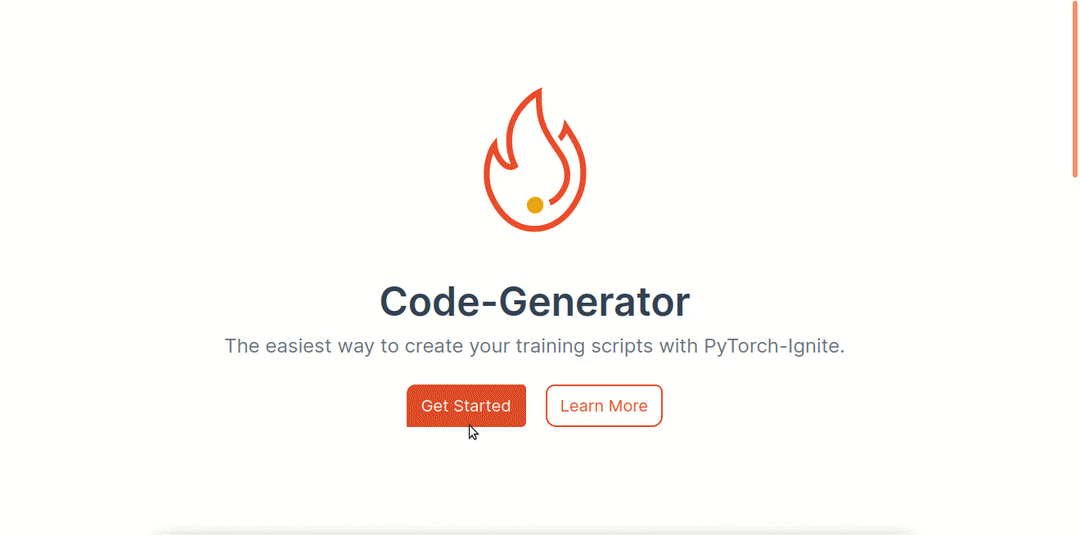https://code-generator.pytorch-ignite.ai/

• What is Code-Generator?: web app to quickly produce quick-start python code for common training tasks in deep learning.

• Why to use Code-Generator?: start working on a task without rewriting everything from scratch.

# Prerequisites

• Basic Python knowledge for working on templates
• Basic HTML/JS knowledge for the web application
• Basic Machine Learning knowledge

.

# How you can help ?

Participating GitHub repositories:

# Prerequisites

• Basic Machine/Deep Learning knowledge
• Basic PyTorch and PyTorch-Ignite knowledge

# Start contributing (2/2)

.

## in this sprint session !

and check out our new website:

https://pytorch-ignite.ai

.

We are looking for contributors to help out with the project.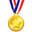Everyone is welcome to contribute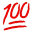.

# The Big Picture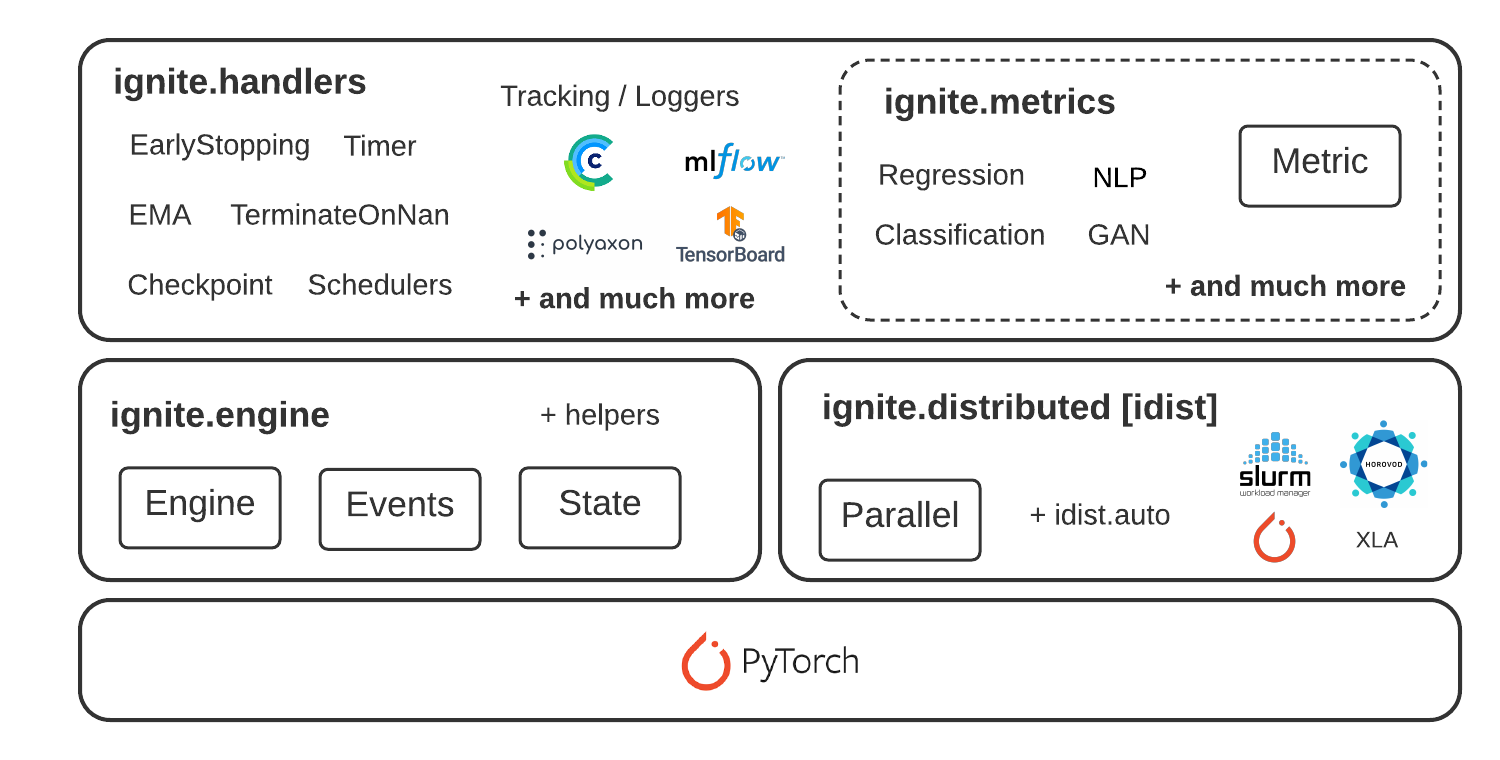## Engine and Event System

 .EngineLoops on user dataApplies an arbitrary user function on batchesEvent systemCustomizable event collectionsTriggers handlers attached to events In its simpliest form:``````fire_event(Events.STARTED) while epoch < max_epochs: fire_event(Events.EPOCH_STARTED) for batch in data: fire_event(Events.ITERATION_STARTED) output = train_step(batch) fire_event(Events.ITERATION_COMPLETED) fire_event(Events.EPOCH_COMPLETED) fire_event(Events.COMPLETED) ``````

### Simplified training and validation loop

No more coding `for/while` loops on epochs and iterations. Users instantiate engines and run them.

``````from ignite.engine import Engine, Events, create_supervised_evaluator
from ignite.metrics import Accuracy

# Setup training engine:
def train_step(engine, batch):
# Users can do whatever they need on a single iteration
# Eg. forward/backward pass for any number of models, optimizers, etc.
# ...

trainer = Engine(train_step)

# Setup single model evaluation engine
evaluator = create_supervised_evaluator(model, metrics={"accuracy": Accuracy()})

def validation():
# print computed metrics
print(trainer.state.epoch, state.metrics)

# Run model's validation at the end of each epoch

# Start the training
``````

### Power of Events & Handlers 🚀

#### 1. Execute any number of functions whenever you wish

Handlers can be any function: e.g. lambda, simple function, class method, etc.

``````trainer.add_event_handler(Events.STARTED, lambda _: print("Start training"))

# attach handler with args, kwargs
mydata = [1, 2, 3, 4]
logger = ...

def on_training_ended(data):
print(f"Training is ended. mydata={data}")
# User can use variables from another scope
logger.info("Training is ended")

# call any number of functions on a single event

@trainer.on(Events.ITERATION_COMPLETED)
def log_something(engine):
print(engine.state.output)
``````

### Power of Events & Handlers

#### 2. Built-in events filtering and stacking

``````# run the validation every 5 epochs
@trainer.on(Events.EPOCH_COMPLETED(every=5))
def run_validation():
# run validation

@trainer.on(Events.COMPLETED | Events.EPOCH_COMPLETED(every=10))
def run_another_validation():
# ...

# change some training variable once on 20th epoch
@trainer.on(Events.EPOCH_STARTED(once=20))
def change_training_variable():
# ...

# Trigger handler with customly defined frequency
@trainer.on(Events.ITERATION_COMPLETED(event_filter=first_x_iters))
# ...

``````

### Power of Events & Handlers

#### 3. Custom events to go beyond standard events

``````from ignite.engine import EventEnum

# Define custom events
class BackpropEvents(EventEnum):
BACKWARD_STARTED = 'backward_started'
BACKWARD_COMPLETED = 'backward_completed'
OPTIM_STEP_COMPLETED = 'optim_step_completed'

def train_step(engine, batch):
# ...
loss = criterion(y_pred, y)
engine.fire_event(BackpropEvents.BACKWARD_STARTED)
loss.backward()
engine.fire_event(BackpropEvents.BACKWARD_COMPLETED)
optimizer.step()
engine.fire_event(BackpropEvents.OPTIM_STEP_COMPLETED)
# ...

trainer = Engine(train_step)
trainer.register_events(*BackpropEvents)

@trainer.on(BackpropEvents.BACKWARD_STARTED)
def function_before_backprop(engine):
# ...
``````

# Out-of-the-box metrics 📈

50+ distributed ready out-of-the-box metrics to easily evaluate models.

• Dedicated to many Deep Learning tasks
• Easily composable to assemble a custom metric
• Easily extendable to create custom metrics
.
``````precision = Precision(average=False)
recall = Recall(average=False)
F1_per_class = (precision * recall * 2 / (precision + recall))
F1_mean = F1_per_class.mean()  # torch mean method
F1_mean.attach(engine, "F1")
``````

# Built-in Handlers

 .Logging to experiment tracking systemsCheckpointing,Early stoppingProfilingParameter schedulingetc. ``````# model checkpoint handler checkpoint = ModelCheckpoint('/tmp/ckpts', 'training') trainer.add_event_handler(Events.EPOCH_COMPLETED(every=2), handler, {'model': model}) # early stopping handler def score_function(engine): val_loss = engine.state.metrics['acc'] return val_loss es = EarlyStopping(3, score_function, trainer) evaluator.add_event_handler(Events.COMPLETED, handler) # Piecewise linear parameter scheduler scheduler = PiecewiseLinear(optimizer, 'lr', [(10, 0.5), (20, 0.45), (21, 0.3), (30, 0.1), (40, 0.1)]) trainer.add_event_handler(Events.ITERATION_STARTED, scheduler) # TensorBoard logger: batch loss, metrics tb_logger = TensorboardLogger(log_dir="tb-logger") tb_logger.attach_output_handler( trainer, event_name=Events.ITERATION_COMPLETED(every=100), tag="training", output_transform=lambda loss: {"batch_loss": loss}, ) tb_logger.attach_output_handler( evaluator, event_name=Events.EPOCH_COMPLETED, tag="training", metric_names="all", global_step_transform=global_step_from_engine(trainer), ) ``````

# Distributed Training support

Run the same code across all supported backends seamlessly

• Backends from native torch distributed configuration: `nccl`, `gloo`, `mpi`
• Horovod framework with `gloo` or `nccl` communication backend
• XLA on TPUs via `pytorch/xla`
``````import ignite.distributed as idist

def training(local_rank, *args, **kwargs):
dataloder_train = idist.auto_dataloder(dataset, ...)

model = ...
model = idist.auto_model(model)

optimizer = ...
optimizer = idist.auto_optimizer(optimizer)

backend = 'nccl'  # or 'gloo', 'horovod', 'xla-tpu' or None
with idist.Parallel(backend) as parallel:
parallel.run(training)
``````

# Distributed Training support

## Distributed launchers

Handle distributed launchers with the same code

• `torch.multiprocessing.spawn`
• `torch.distributed.launch`
• `horovodrun`
• `slurm`

# Distributed Training support

## Unified Distributed API

• High-level helper methods

• `idist.auto_model()`
• `idist.auto_optim()`
• `idist.auto_dataloader()`
• Collective operations

• `all_reduce`, `all_gather`, and more

Any questions before we go on ?